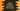# Python Conditional Statements : Python Tutorial 13## Python Conditional Statements :

If - else statements are known as conditional statements. In simple words, if the condition defined for “if” block is true, then the block of statements defined for if block will execute. Otherwise, the code defined for “else” block will execute.

As we have seen that the indentation is used in python instead of curly braces or anything, in case of if-else statements also all lines after “if” statement with same indentation will run for “if” condition  . Same thing for “else” block as well.

Any non-zero values will be interpreted as “True” in python except “0” and “None” .

``````if True:
print "True for True"
else:
print "False for True"

if False:
print "True for False"
else:
print "False for False"

if 1:
print "True for 1"
else:
print "False for 1"

if 'a':
print "True for a"
else:
print "False for a"

if "False":
print "True for \"False\" "
else:
print "False for \"False\" "``````

The output will be :

``````True for True
False for False
True for 1
True for a
True for "False”``````

Let’s try if-else statements with some mathematical expressions :

``````if 10 > 1:
print "10 is greater than 1"
else:
print "error !!!"

i = 20

if i%5 == 0:
print "True"
else:
print "False"
print "This line is not in else case”``````

It will print :

``````10 is greater than 1
True
This line is not in else case``````

One thing we have noticed in the above example that the last line is written just below “print “Failed”” line but since its indentation is different , it will not considered with the “else” case lines.

## If .. Else if … Else :

If ,ElseIf, Else contains three blocks. First the condition for if block is checked. If it is true, “if” block will be executed and control will exit . If false, it will check condition on “else if” block. If “else if “ condition is also false, finally it will check “else” condition. “else if” is denoted as “elif” in python. Let’s take a look into the following example :

``````i = 100

if i > 101:
print "Inside if"
elif i > 90:
print "Inside elif"
else:
print "Inside else"``````

Here, the output will be “Inside elif”. You can try this program with different “i” values for more clear understanding.

## Nesting of statements :

We can put if, else or elif statements inside other if,else or elif statements . This is called nesting of statements. Check the below example :

``````i = 100

if i > 90:
if i > 100:
print "i is greater than 100"

elif i < 100:
print "i is less than 100"

else:
print "i is 100”``````

It will print “i is 100”.  The only way you can find a nested if - else is the indentation.